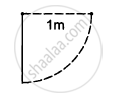Advertisement Remove all ads

# A Metre Stick Weighing 240 G is Pivoted at Its Upper End in Such a Way that It Can Freely Rotate in a Vertical Place Through this End (See the Following Figure). - Physics

Sum

A metre stick weighing 240 g is pivoted at its upper end in such a way that it can freely rotate in a vertical place through this end (see the following figure). A particle of mass 100 g is attached to the upper end of the stick through a light string of length 1 m. Initially, the rod is kept vertical and the string horizontal when the system is released from rest. The particle collides with the lower end of the stick and sticks there. Find the maximum angle through which the stick will rise.Advertisement Remove all ads

#### Solution

Let the angular velocity of stick after the collision be ω.

Given

Mass of the stick = m = 240 g

Mass of the particle = m' = 100 g

Length of the string (or rod) = r = l = 1 m

Moment of inertia of the particle about the pivoted end = $I' = m' r^2$

$\Rightarrow I' = \left( 0 . 1 \times 1^2 \right)$

$\Rightarrow I' = 0 . 1 kg - m^2$

Moment of inertia of the rod about the pivoted end = $I = \frac{m l^2}{3}$

$\Rightarrow I = \left( \frac{0 . 24}{3} \times 1^2 \right)$

$\Rightarrow I = 0 . 08 kg - m^2$

Applying the law of conservation of energy, we get

Final energy of the particle = Initial energy of the rod

$mgh = \frac{1}{2}I \omega^2$

$\Rightarrow \frac{1}{2}I \omega^2 = 0 . 1 \times 10 \times 1$

$\Rightarrow \omega = \sqrt{20}$

For collision, we have

$0 . 1 \times 1^2 \times \sqrt{20} + 0$

$= \left[ \left( \frac{0 . 24}{3} \right) \times 1^2 + \left( 0 . 1 \right)^2 \left( 1 \right)^2 \right] \omega$

$\Rightarrow \omega = \frac{\sqrt{20}}{\left[ 10 \times \left( 0 . 18 \right) \right]}$

$\Rightarrow 0 - \frac{1}{2}I \omega^2 = - m_1 gl\left( 1 - \cos \theta \right) - m_2 g\frac{1}{2} \left( 1 - \cos \theta \right)$

$\Rightarrow \frac{1}{2} I \omega^2 = - mgl \left( 1 - \cos \theta \right) - m_2 g\frac{1}{2} \left( 1 - \cos \theta \right)$

$\Rightarrow \frac{1}{2}I \omega^2 = 0 . 1 \times 10 \left( 1 - \cos \theta \right) - 0 . 24 \times 10 \times 0 . 5 \left( 1 - \cos \theta \right)$

$\Rightarrow \frac{1}{2} \times 0 . 18 \times \left( \frac{20}{324} \right) = 2 . 2 \times 9 \left( 1 - \cos \theta \right)$

$\Rightarrow \left( 1 - \cos \theta \right) = \frac{1}{\left( 2 . 2 \times 1 . 8 \right)}$

$\Rightarrow 1 - \cos \theta = 0 . 252$

$\Rightarrow \cos \theta = 1 - 0 . 252 = 0 . 748$

$\Rightarrow \theta = \cos^{- 1} \left( 0 . 748 \right) = 41^\circ$

Concept: Values of Moments of Inertia for Simple Geometrical Objects (No Derivation)
Is there an error in this question or solution?
Advertisement Remove all ads

#### APPEARS IN

HC Verma Class 11, 12 Concepts of Physics 1
Chapter 10 Rotational Mechanics
Q 68 | Page 199
Advertisement Remove all ads
Advertisement Remove all ads
Share
Notifications

View all notifications

Forgot password?